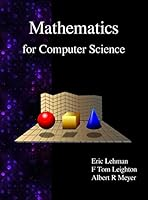# Mathematics for Computer Science## Book Description

This book covers elementary discrete for computer science and engineering. It emphasizes mathematical definitions and proofs as well as applicable methods. Topics include formal notation, proof methods; induction, well-ordering; sets, relations; elementary graph ; integer congruences; asymptotic notation and growth of ; permutations and combinations, counting principles; discrete probability. Further selected topics may also be covered, such as recursive definition and structural induction; state machines and invariants; recurrences; generating .

Part 1 Proofs
Chapter 1 What is Proof
Chapter 2 Well Ordering Principle
Chapter 3 Logical Formulas
Chapter 4 Mathematical Data Types
Chapter 5 Induction
Chapter 6 State Machines
Chapter 7 Recursive Data Types
Chapter 8 Inﬁnite Sets

Part 2 Structures
Chapter 9
Chapter 10 Directed & Partial Orders
Chapter 11 Communication Networks
Chapter 12 Simple Graphs
Chapter 13 Planar Graphs

Part 3 Counting
Chapter 14 Sums & Asymptotics
Chapter 15 Cardinality Rules
Chapter 16 Generating Functions

Part 4 Probability
Chapter 17 Events & Probability Spaces
Chapter 18 Conditional Probability
Chapter 19 Random Variables
Chapter 20 Deviation from the Mean
Chapter 21 Random Walks

Part 5 Recurrences
Chapter 22 Recurrences